In focus

# SQL Functions

## This article describes SQL Functions. SQL has many built-in functions for performing operations on numeric or string values.

Avinash Thakur Apr 04, 2016

SQL has many built-in functions for performing operations on numeric or string values. The following is the list of all useful SQL functions with examples:

• COUNT ():  It is used to returns the number of rows.

• AVG (): It is used to returns the average value.

• MAX (): It is used to returns the largest value.

• MIN (): It is used to returns the smallest value.

• SUM (): It is used to returns the sum.

• LEN (): It is used to returns the length of a text field.

• UPPER (): It is used to converts a field to upper case.

• LOWER (): It is used to converts a field to lower case.

• GETDATE (): It returns the current date and time from the server's system clock.

• DATEDIFF(): It is used to compare and return the difference between date items such as days, weeks, minutes, and hours.
AVG () Function: This function returns the average value of a numeric column.

Syntax: SELECT AVG (column_name) FROM table_name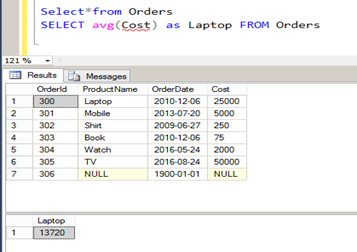COUNT () Function: This function returns the number of rows that match a specified criteria.

Syntax: SELECT COUNT(column_name) FROM table_name;MAX () Function: This function returns the largest value of the selected column.

Syntax: SELECT MAX (column_name) FROM table_name;MIN () Function: This function returns the smallest value of the selected column.

Syntax: SELECT MIN (column_name) FROM table_name;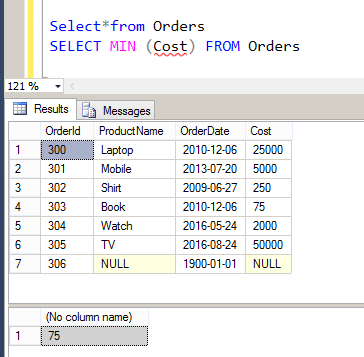SUM () Function: This function returns the total sum of a numeric column.

Syntax: SELECT SUM(column_name) FROM table_name;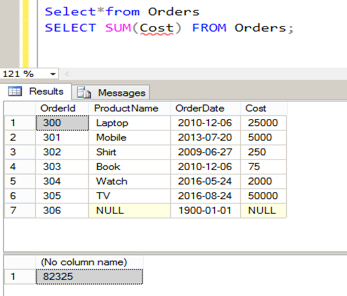GROUP BY : This statement is used in conjunction with the aggregate functions to group the result-set by one or more columns.

Syntax:  SELECT column_name, aggregate_function(column_name) FROM table_name WHERE column_name operator value GROUP BY column_name;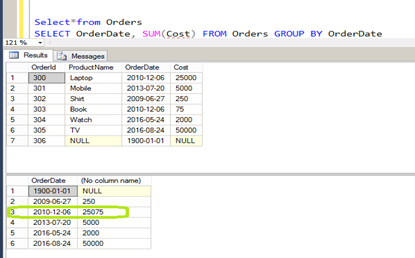UCASE () Function: This function converts the value of a field to uppercase.

Syntax: SELECT UPPER (column_name) FROM table_name;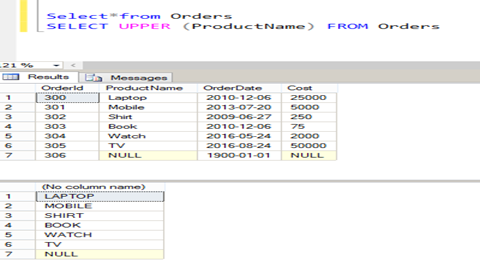LCASE() Function: This function converts the value of a field to lowercase.

Syntax: SELECT LOWER(column_name) FROM table_name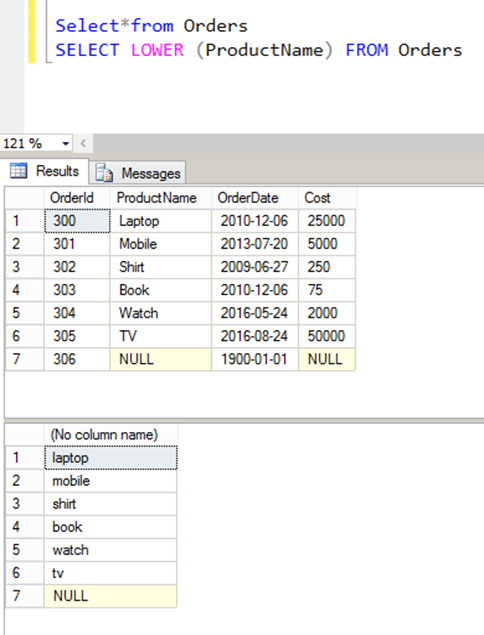LEN() Function: This function returns the length of the value in a text field.

Syntax: SELECT LEN(column_name) FROM table_name;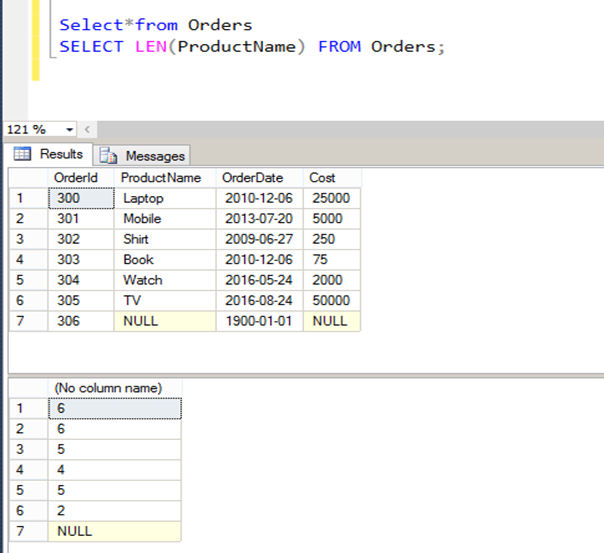GETDATE() Function: This function return the current time from the server’s system time.

Syntax: select getdate();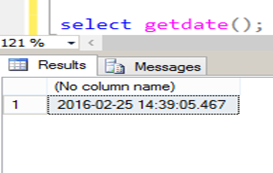DATEDIFF () Function: This function is use to compare and return the difference between date items such as days ,weeks, minutes.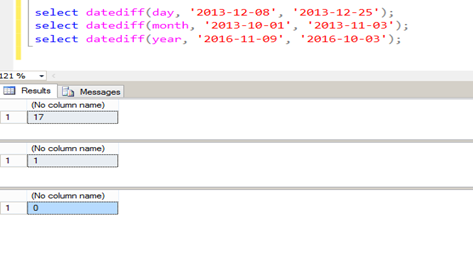Here's a free e-book on SQL Server 2014: Introducing Microsoft SQL Server 2014